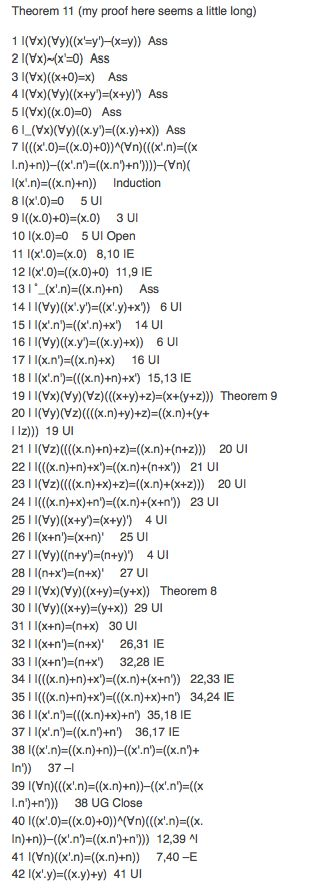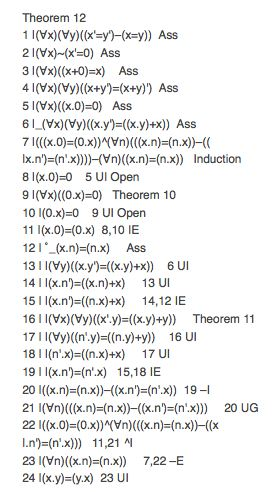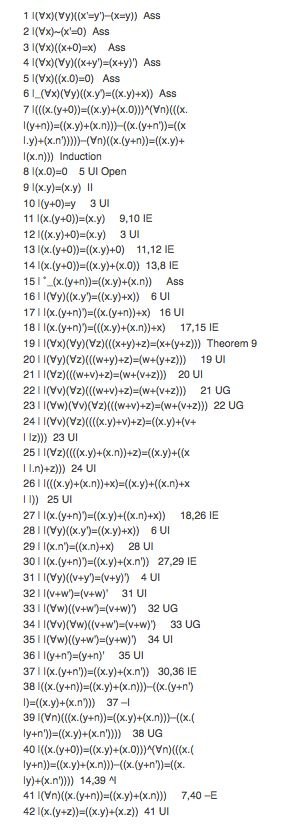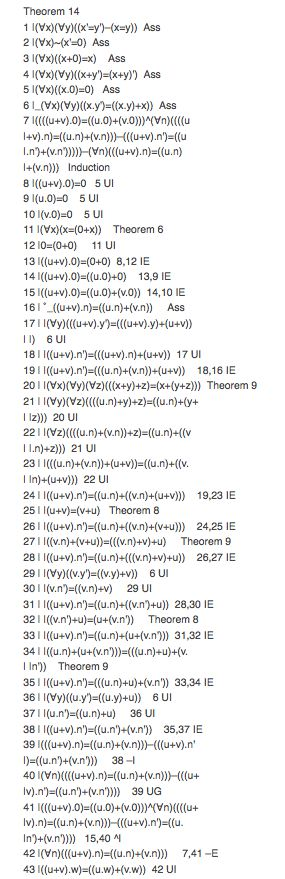# Tutorial 5: Number Theory II

Logical System

## Tutorial 5: Number Theory II

Formal Number Theory. Just more theorems for you.

### Recall

Formal Number Theory has five proper symbols {=,',+, .,0} and six proper axioms

(∀x)(∀y)(x'=y'⊃x=y),
(∀x)~(x'=0),
(∀x)(x+0=x),
(∀x)(∀y)(x+y'=(x+y)'),
(∀x)(x.0=0),
(∀x)(∀y)(x.y'=x.y+x)

And the axiom (metalanguage) schema of induction. If φ[n] is any formula in the object language with free variable n then

(φ∧(∀n)(φ[n]⊃φ[n'])) ⊃ (∀n)φ[n]

is an axiom. Actually, it is usual to take the closure of this as an axiom (that is, all free variables are quantified over). However, since we have no free variables in the axioms we can form the closure by Universal Generalization, if we want it.

Derive the following theorems (solutions are provided at the end of this Journal)

## Exercise to accompany Number Theory II.

exercises under construction 12/14/06

Derive the following theorems (solutions are provided at the end of this Journal)

### Exercise 1(of 3)

Derive the following

Theorem 11

(∀x)(∀y)(x'=y'⊃x=y),
(∀x)~(x'=0),
(∀x)(x+0=x),
(∀x)(∀y)(x+y'=(x+y)'),
(∀x)(x.0=0),
(∀x)(∀y)(x.y'=x.y+x)

x'.y=x.y+y

Theorem 12

(∀x)(∀y)(x'=y'⊃x=y),
(∀x)~(x'=0),
(∀x)(x+0=x),
(∀x)(∀y)(x+y'=(x+y)'),
(∀x)(x.0=0),
(∀x)(∀y)(x.y'=x.y+x)

x.y=y.x

Theorem 13 (distributivity)

(∀x)(∀y)(x'=y'⊃x=y),
(∀x)~(x'=0),
(∀x)(x+0=x),
(∀x)(∀y)(x+y'=(x+y)'),
(∀x)(x.0=0),
(∀x)(∀y)(x.y'=x.y+x)

x.(y+z)=x.y+x.z

Theorem 14 (distributivity)

(∀x)(∀y)(x'=y'⊃x=y),
(∀x)~(x'=0),
(∀x)(x+0=x),
(∀x)(∀y)(x+y'=(x+y)'),
(∀x)(x.0=0),
(∀x)(∀y)(x.y'=x.y+x)

(u+v).w=u.w+v.w

### Exercise 2(of 3)

Theorem 15 (associativity of .)
(∀x)(∀y)(x'=y'⊃x=y),
(∀x)~(x'=0),
(∀x)(x+0=x),
(∀x)(∀y)(x+y'=(x+y)'),
(∀x)(x.0=0),
(∀x)(∀y)(x.y'=x.y+x)

(u.v).w=u.(v.w)

Theorem 16
(∀x)(∀y)(x'=y'⊃x=y),
(∀x)~(x'=0),
(∀x)(x+0=x),
(∀x)(∀y)(x+y'=(x+y)'),
(∀x)(x.0=0),
(∀x)(∀y)(x.y'=x.y+x)

(u+v=w+v)⊃u=w

For the next few theorems we will let 1 be an abbreviation for 0' (we do this by adding 1=0' as an axiom (premise or definition)) and similarly for 2 which abbreviates 0''.

Theorem 17
1=0',
(∀x)(∀y)(x'=y'⊃x=y),
(∀x)~(x'=0),
(∀x)(x+0=x),
(∀x)(∀y)(x+y'=(x+y)'),
(∀x)(x.0=0),
(∀x)(∀y)(x.y'=x.y+x)

u+1=u'

Theorem 18
1=0' ,
(∀x)(∀y)(x'=y'⊃x=y),
(∀x)~(x'=0),
(∀x)(x+0=x),
(∀x)(∀y)(x+y'=(x+y)'),
(∀x)(x.0=0),
(∀x)(∀y)(x.y'=x.y+x)

u.1=u

### Exercise 3(of 3)

Theorem 19
1=0',
(∀x)(∀y)(x'=y'⊃x=y),
(∀x)~(x'=0),
(∀x)(x+0=x),
(∀x)(∀y)(x+y'=(x+y)'),
(∀x)(x.0=0),
(∀x)(∀y)(x.y'=x.y+x)

u+1=u'

Theorem 20
2=0'',
(∀x)(∀y)(x'=y'⊃x=y),
(∀x)~(x'=0),
(∀x)(x+0=x),
(∀x)(∀y)(x+y'=(x+y)'),
(∀x)(x.0=0),
(∀x)(∀y)(x.y'=x.y+x)

2.u=u+u

### Solutions

Theorem 11 (my proof here seems a little long)Theorem 13. This proof is probably long, but it also involves two changes of variables due to wishing to substitute a term like y.n for x in (∀x)(∀y)<something> by UI and, of course, that is forbidden because of capturing. This convinces me that we would do better to state our theorems in terms of variables not quantified in the axioms, u, v, w, say.